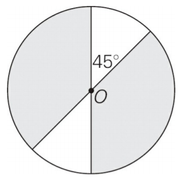## In the figure above, what fraction of the circle with center

##### This topic has expert replies
Legendary Member
Posts: 920
Joined: 01 Mar 2018
Followed by:2 members

### In the figure above, what fraction of the circle with center

by Gmat_mission » Mon Jun 10, 2019 5:24 am

00:00

A

B

C

D

E

## Global StatsIn the figure above, what fraction of the circle with center O is shaded?

A. 1/8
B. 1/4
C. 2/3
D. 3/4
E. 5/6

[spoiler]OA=D[/spoiler]

Source: Princeton Review

### GMAT/MBA Expert

GMAT Instructor
Posts: 2094
Joined: 04 Dec 2012
Thanked: 1443 times
Followed by:245 members
by ceilidh.erickson » Tue Jun 11, 2019 11:50 am
Angles opposite one another have the same measurement. Thus, both non-shaded regions measure 45 degrees from the center of the circle, for a total of 90 degrees.

A circle is 360 degrees, so 90 degrees is 1/4 of the circle that is unshaded. That leaves 3/4 of the circle shaded.

Ceilidh Erickson
EdM in Mind, Brain, and Education

### GMAT/MBA Expert

GMAT Instructor
Posts: 5676
Joined: 25 Apr 2015
Location: Los Angeles, CA
Thanked: 43 times
Followed by:24 members
by [email protected] » Tue Jun 11, 2019 7:15 pm
Gmat_mission wrote:In the figure above, what fraction of the circle with center O is shaded?

A. 1/8
B. 1/4
C. 2/3
D. 3/4
E. 5/6

[spoiler]OA=D[/spoiler]

Source: Princeton Review
Since the central angle of each white region is 45 degrees, the central angle of each shaded region is 180 - 45 = 135. Thus, (135 + 135)/360 = 270/360 = 3/4 is shaded.

Alternate Solution:

A sector corresponding to 45Â° will have an area which is 1/8 of the complete circle. The unshaded region consists of two such sectors; therefore, the unshaded region is 1/8 + 1/8 = 1/4 of the whole circle. Thus, the shaded region is 1 - 1/4 = 3/4 of the whole circle.